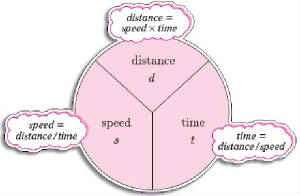Home Physics Chemistry Physical Science Physical Science Unit 1 Physical Science Unit 2 Earth Science Math for Science Documents Quiz Links Free-Write

Motion

A.    Recognizing Motion

1.      An object is in motion when its distance from another object is changing.

2.      Movement is based upon ones point of view based on a reference point.

a.      We assume reference points are stationary.

b.      A reference point is a place or object used for comparison to determine if something is in motion.

c.      An object is in motion if it changes position relative to a reference point.

B.     Describing Distance

1.      In science we use the International System of Units (SI) and is based on units of 10.

a.      The basic SI unit of length is a meter (m).

b.      cm = centimeter: 1/100 of a meter

c.      mm = millimeter:  1/1000 of a meter

d.      km = kilometer: 1000 meters

C.     Calculating Speed

1.      Speed is a type of rate.2.      The speed of an object is the distance the object travels per unit of time.

3.      Formula: Speed =  Distance / Time

4.      When the speed of an object does not change, it is known as Constant Speed.

5.      Constant speed is difficult to maintain. (bike, car, running, walking)

6.      Average speed (most common) is calculated when constant speeds are not or can not be maintained.

D.    Velocity

1.      When you know both the speed and direction of an object’s motion, you know the velocity of the object.

2.      Speed in a given direction is called velocity.

E.    Acceleration

1.    Acceleration is the rate at which velocity changes.

a.  Velocity has 2 components: speed and direction.

2.    Acceleration changes if

a.  speed increases

b.  speed decreases (sometimes deceleration, or negative acceleration)

c.  direction changes.

3.    To determine the acceleration of an object, you must calculate the change in velocity during a unit of time.

a. If velocity is measured in meters / second and time is measured in seconds, the unit of acceleration is meters per second per second.

b. This unit is written as m/s². (meters per second squared)

4.    To calculate the acceleration, subtract the initial velocity from the final velocity and divide that by the    elapsed time.

(Final velocity – initial velocity)     (Vf – Vi)
-----------------------------------  =  ---------  =  Acceleration
(Final time - initial time)         (tf – ti)

F.     Graphing Motion

1.     A motion graph indicates time (x-axis) and distance (y-axis).

2.     The slope of the line on a motion (position) graph represents speed.

a. If linear, the speed is constant, or unchanging.

b. If the line has more than one slope, the speed changes.

c. If the line is a curve, the speed is uniformly changing (acceleration).